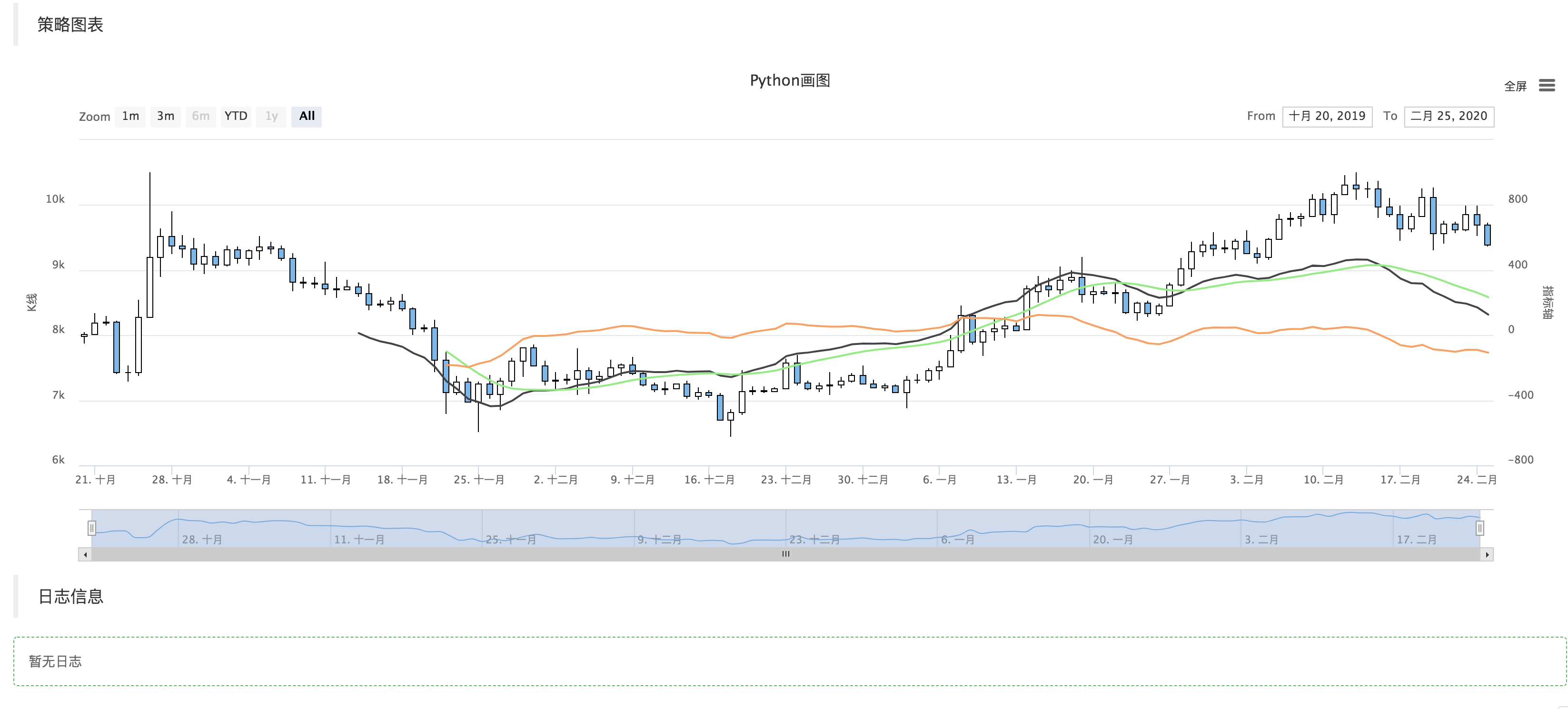# Python版MACD画图范例

Author: 小小梦, Created: 2020-02-27 19:18:17, Updated: 2020-03-03 23:38:14

## Python版MACD画图范例

``````'''backtest
start: 2020-01-28 00:00:00
end: 2020-02-26 00:00:00
period: 1d
exchanges: [{"eid":"OKEX","currency":"BTC_USDT"}]
'''

ChartCfg = {
'__isStock': True,
'title': {
'text': 'Python画图'
},
'yAxis': [{
'title': {'text': 'K线'},
'style': {'color': '#4572A7'},
'opposite': False
}, {
'title': {'text': '指标轴'},
'opposite': True
}],
'series': [{
'type': 'candlestick',
'name': '当前周期',
'id': 'primary',
'data': []
}, {
'type': 'line',
'id': 'dif',
'name': 'DIF',
"yAxis" : 1,
'data': []
}, {
'type': 'line',
'id': 'dea',
'name': 'DEA',
"yAxis" : 1,
'data': []
}, {
'type': 'line',
'id': 'macd',
'name': 'MACD',
"yAxis" : 1,
'data': []
}]
}

def main():
global ChartCfg
preTime = 0
chart = Chart(ChartCfg)
chart.reset()
while True:
while True:
r = _C(exchange.GetRecords)
if len(r) > 50:
break
# 计算指标
macd = TA.MACD(r)

LogStatus(_D(), len(r))

# 画图
for i in range(len(r)):
if r[i]["Time"] == preTime:
chart.add(0, [r[i]["Time"], r[i]["Open"], r[i]["High"], r[i]["Low"], r[i]["Close"]], -1)
elif r[i]["Time"] > preTime:
chart.add(0, [r[i]["Time"], r[i]["Open"], r[i]["High"], r[i]["Low"], r[i]["Close"]])
preTime = r[i]["Time"]
Sleep(500)

``````

### 可以直接回测Related

More